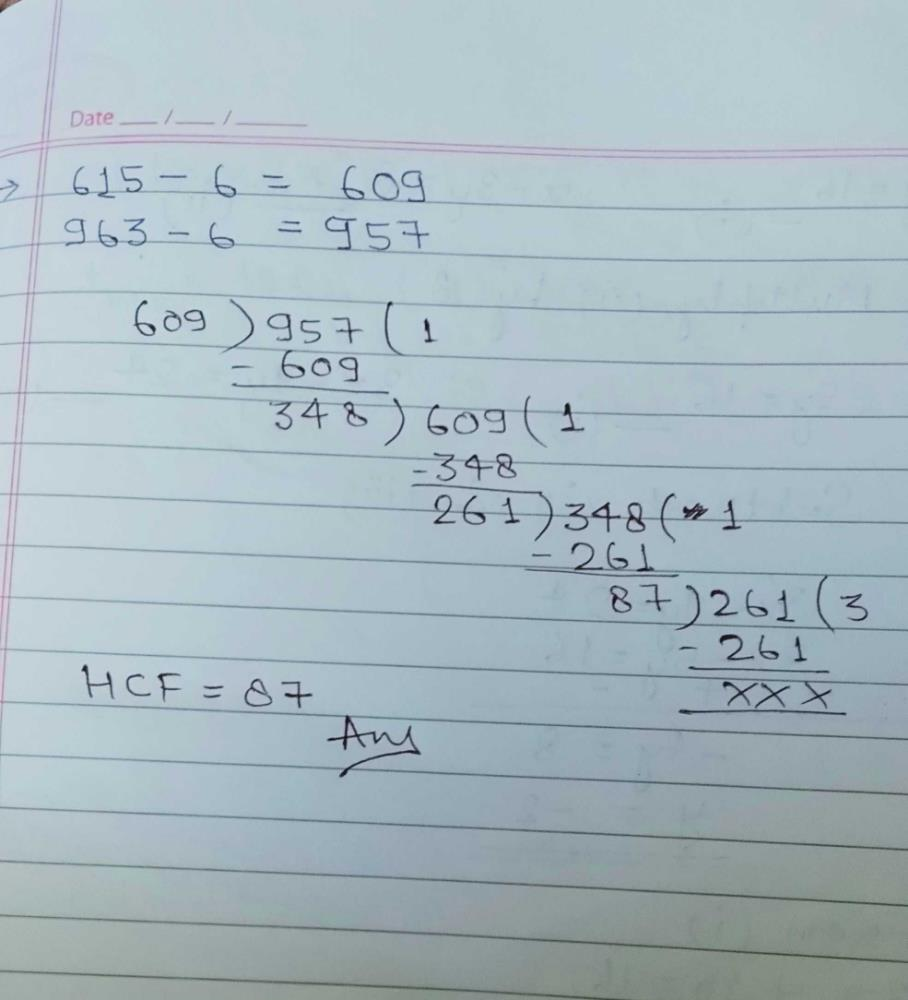Courses

# The largest number which divides 615 and 963 leaving remainder 6 in each case isa)82b)95c)87d)93Correct answer is option 'C'. Can you explain this answer? Related Test: MCQ : Real Numbers - 2

## Class 10 Question

By Karthik Varma · May 18, 2020 ·Class 10
Nirmal Kumar answered Apr 10, 2020
According to the question the new numbers are--
615-6=609,
and 963-6=957,
since , 957>609,
by euclid's division Lemma-->
957=609×1+348,
the reminder is not zero we apply the process again,
609=348×1+261,
is not equal to zero so, by applying the process again
348=261×1+87,
261=87×3+0,
since r=0 list of the process and get
the no.=87

Sarita Pandey answered May 10, 2020Diana Nair answered May 18, 2020
Correct option is C
Here largest number means HCF OF 615 and 963
963 - 6 = 957
615 - 6 = 609
957 = 609 × 1 + 348
609 = 348 × 1 + 261
348 = 261 × 1 + 87
261 = 87 × 3 + 0
So the HCF of 615 and 963 is 87.